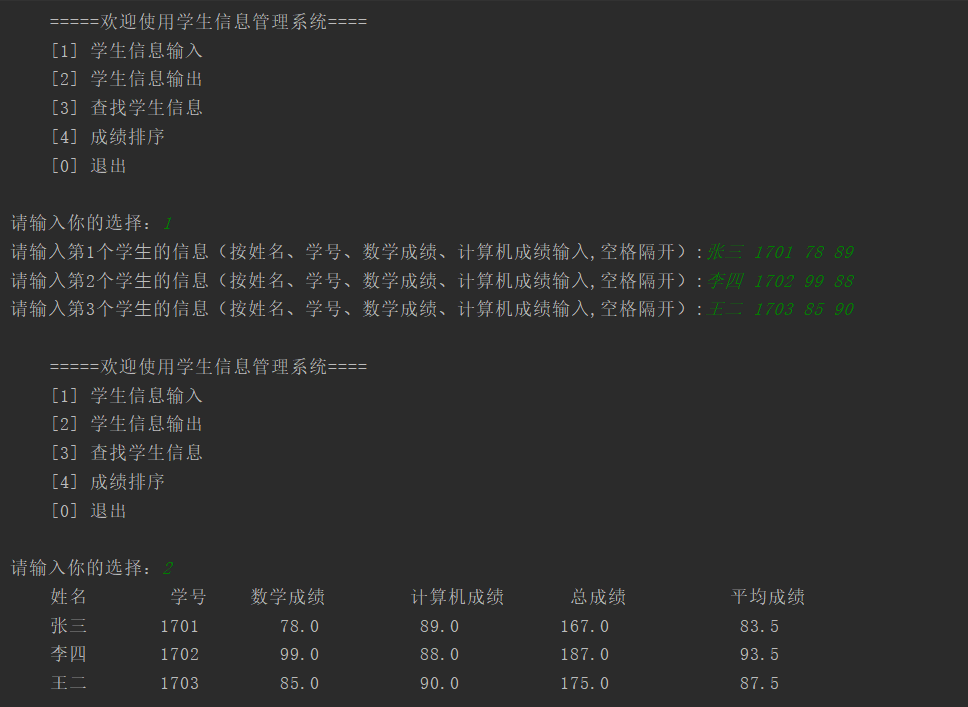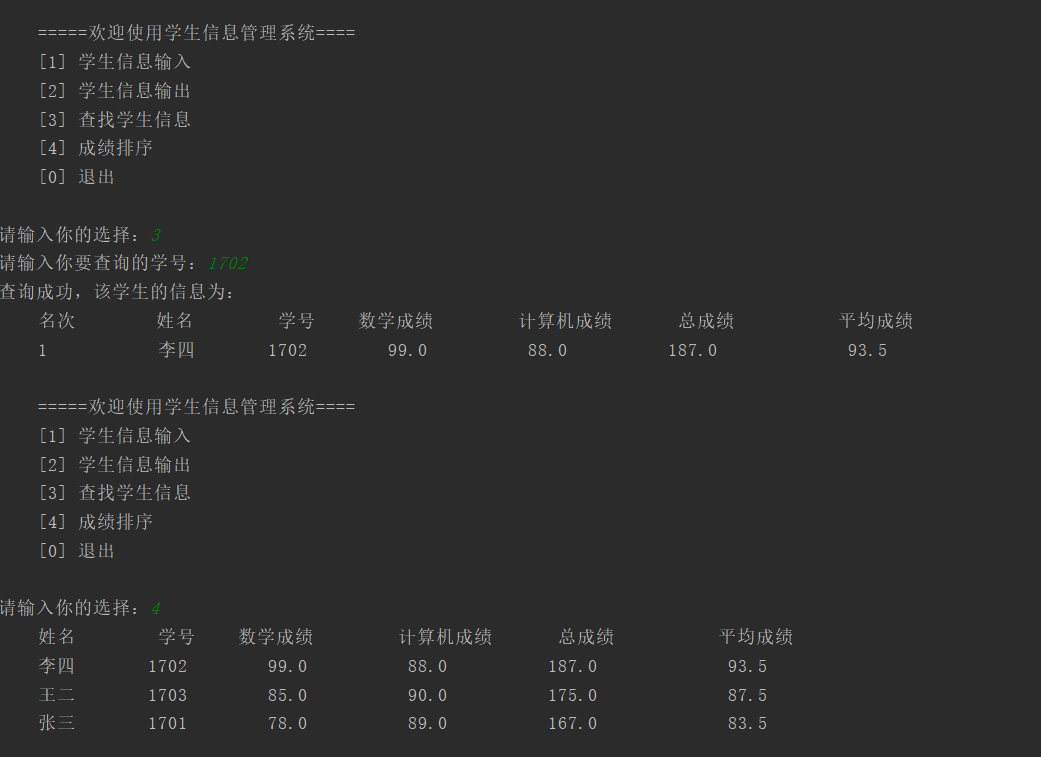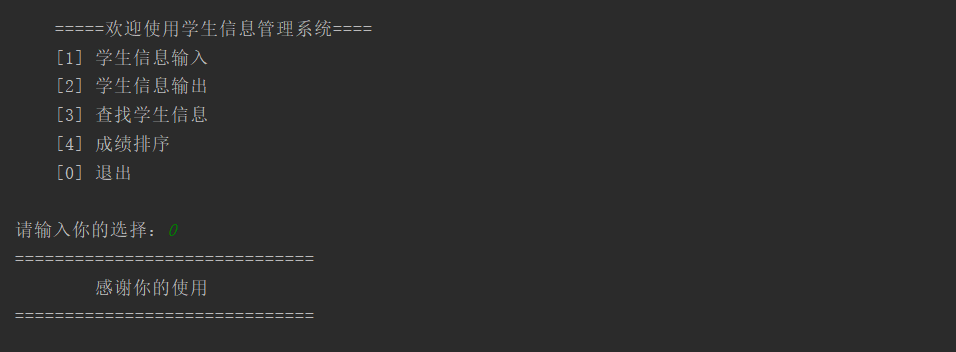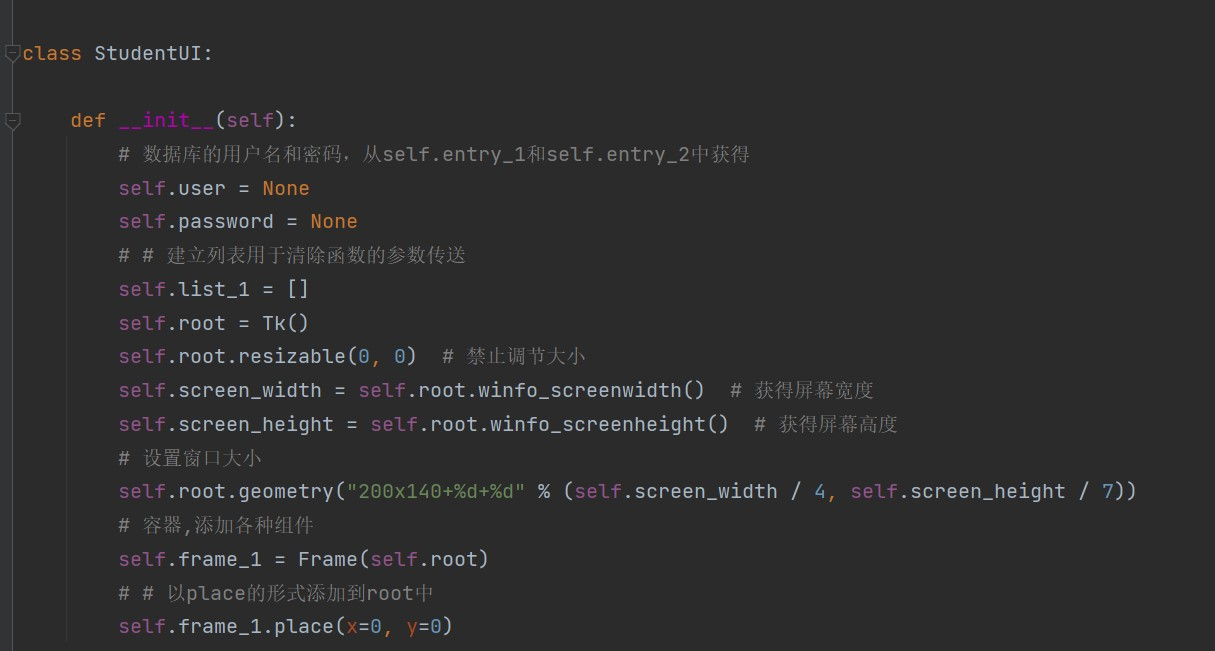• 主要为大家详细介绍了python学生信息管理系统，具有一定的参考价值，感兴趣的小伙伴们可以参考一下
• 主要为大家详细介绍了python学生信息管理系统的完整版本代码，具有一定的参考价值，感兴趣的小伙伴们可以参考一下
• python编写的学生信息管理系统，GUI界面。实现了增删改查的基本功能和保存为TXT文件，详情请看我的博客 https://blog.csdn.net/qq_52889967/article/details/118967084
• 本文为大家分享了python学生信息管理系统的初级实现代码，供大家参考，具体内容如下 学生信息管理项目，要求带操作界面，并完成每项操作： ＋－－－－－－－－－－－－－－－－－－－－－－＋ ｜　1）添加学生信息　...
• 主要介绍了python学生信息管理系统的实现代码，代码简单，复制即可使用,需要的朋友可以参考下
• 主要为大家详细介绍了python学生信息管理系统，具有一定的参考价值，感兴趣的小伙伴们可以参考一下
• python编写的学生信息管理系统+MySQL，适合刚学python的新手
• 继上篇博客Python实现简易通讯录后，我就想写一个复杂点的学生信息管理系统，这次实现的功能有 1.学生信息的录入管理； 　2.学生选课操作； 　3.学生选课情况查询； 这次仍然用到sqlite3模块。虽然看着挺简单，...
• 下面通过python实现一个简单的学生信息管理系统 stuInfo=[] def main(): while True: printMenu() #打印菜单 key=int(input('请输入功能对应的数字：')) if key==1: addInfo() #添加学生信息 elif key==2: ...
• 主要介绍了Python实现GUI学生信息管理系统，具有一定的参考价值，感兴趣的小伙伴们可以参考一下
• 设计一个GUI界面的学生信息管理系统，方便保存学生的部分信息。 有五类功能： 1、 增加学生信息：输入姓名、学号、年龄、手机号 2、 修改学生信息：输入新的姓名、学号、年龄、手机号 3、 删除学生信息：以学号为...
• #菜单 def meun(): menu_info = '''＋－－－－－－－－－－－－－－－－－－－－－－＋ ｜ １）添加学生信息 ｜ ｜ ２）显示所有学生的信息 ｜ ｜ ３）删除学生信息 ｜ ｜ ４）修改学生信息 ...
#菜单
def meun():
｜ １）添加学生信息                         ｜
｜ ２）显示所有学生的信息                    ｜
｜ ３）删除学生信息                         ｜
｜ ４）修改学生信息                         ｜
｜ ５）按学生成绩高－低显示学生信息           ｜
｜ ６）按学生成绩低－高显示学生信息           ｜
｜ ７）按学生年龄高－低显示学生信息           ｜
｜ ８）按学生年龄低－高显示学生信息           ｜
｜ ９）保存学生信息到文件（students.txt)     ｜
｜ １０）从文件中读取数据（students.txt)     ｜
｜ 退出：其他任意按键＜回车＞                ｜
＋－－－－－－－－－－－－－－－－－-－－－－－＋
'''
#｜１）添加学生信息
l=[]
while True:
name=input('请输入学生名字: ')
if not name:
print('~~~输入完毕~~~')
break
try:
age=int(input('学生年龄: '))
score=int(input('学生成绩: '))
except:
print('输入错误,请重新输入')
continue
lst={'name':name,'age':age,'score':score}
l.append(lst)
return l

# ｜２）显示所有学生的信息
def show_student_info(student_info,del_name=''):
if not student_info:
pritn('无学生信息,返回菜单')
return
# center()返回一个原字符串居中, 并使用空格填充至长度width的新字符串。默认填充字符为空格
print('姓名'.center(8), '年龄'.center(4), '成绩'.center(4))
for i in student_info:
print(i.get('name').center(10),str(i.get('age')).center(4),str(i.get('score')).center(8))

# ｜３）删除学生信息
def del_student_info(student_info,del_name=''):
if not del_name:
del_name = input("请输入删除的学生姓名：")
for info in student_info:
if del_name == info.get("name"):
return info
raise IndexError("学生信息不匹配,没有找到%s" %del_name)

# ｜４）修改学生信息
def mod_student_info(student_info):
mod_name=input('请输入要修改的学生名称:')
for info in student_info:
if info.get('name')==mod_name:
mod_age=int(input('请输入学生年龄:'))
mod_score=int(input('请输入学生成绩:'))
info={'name':mod_name,'age':mod_age,'score':mod_score}
return info
raise IndexError('姓名不匹配:%s'%mod_name)

#用于年龄排序:
def get_age(*l):
for x in l:
return x.get('age')

#用于成绩排序:
def get_score(*l):
for x in l:
return x.get('score')

from operator import itemgetter

# ｜５）按学生成绩高－低显示学生信息
def score_reduce(student_info):
print('按学生成绩高－低显示')
#reverse -- 排序规则，reverse = True 降序 ， reverse = False 升序（默认)
mit=sorted(student_info,key=get_score,reverse=True)
show_student_info(mit)

# ｜６）按学生成绩低－高显示学生信息
def score_rise(student_info):
print("按学生成绩低－高显示")
mit=sorted(student_info, key=get_score())

# ｜７）按学生年龄高－低显示学生信息
def age_reduce(student_info):
print("按学生年龄高－低显示：")
mit = sorted(student_info ,key = get_age,reverse = True)
show_student_info(mit)

# ｜８）按学生年龄低－高显示学生信息
def age_rise(student_info):
print("按学生年龄低－高显示：")
mit = sorted(student_info ,key = get_age)
show_student_info(mit)

# ｜９）保存学生信息到文件（students.txt)
def save_info(student_info):
try:
# 以写模式打开，并清空文件内容
student_txt = open('student.txt','w')
except Exception as e:
# 文件不存在，创建文件并打开
student_txt = open('student.txt','x')
for info in student_info:
student_txt.write(str(info)+'\n')
student_txt,close()

# ｜10）从文件中读取数据（students.txt)
old_info = []
try:
students_txt = open("students.txt")
except:
print("暂未保存数据信息")  # 打开失败，文件不存在说明没有数据保存
return
while True:
if not info:
break
# print(info)
info = info.rstrip()  # 去掉换行符
# print(info)
info = info[1:-1]  # 去掉｛｝
# print(info)
student_dict = {}  # 单个学生字典信息
for x in info.split(","):  # 以，为间隔拆分
# print(x)
key_value = []  # 开辟空间，key_value存key,key_value存value
for k in x.split(":"):  # 以：为间隔拆分
k = k.strip()  # 去掉首尾空字符
# print(k)
if k == k[-1] and len(k) > 2:  # 判断是字符串还是整数
key_value.append(k[1:-1])  # 去掉　首尾的＇
else:
key_value.append(int(k))
# print(key_value)
student_dict[key_value] = key_value  # 学生信息添加
# print(student_dict)
old_info.append(student_dict)  # 所有学生信息汇总
students_txt.close()
return old_info

def main():
student_info=[]
while True:
meun()
number=input('请输入您的选项: ')
if number == '1':

elif number == '2':
show_student_info(student_info)

elif number == '3':
try:
student_info.remove(del_student_info(student_info))
except Exception as e:
print(e)

elif number == '4':
try:
student=mod_student_info(student_info)
except Exception as e:
print(e)
else:
# 首先按照根据输入信息的名字，从列表中删除该生信息，然后重新添加该学生最新信息
student_info.remove(del_student_info(student_info, del_name=student.get("name")))
student_info.append(student)

elif number == '5':
score_reduce(student_info)

elif number == '6':
score_rise(student_info)

elif number == '7':
age_reduce(student_info)

elif number == '8':
age_rise(student_info)

elif number == '9':
save_info(student_info)

elif number == '10':

else:
break

input('--回车返回菜单--')

main()

展开全文• 大学期间写的一份Python学生信息管理系统，使用的是文件存储的格式，但是没有学习数据库之类的东西，只能这样写了。代码包含：教师端学生端的登录，学生基本信息的增删改查等四项基本功能。教师端功能全，学生端把...
• 主要为大家详细介绍了python实现简易学生信息管理系统，具有一定的参考价值，感兴趣的小伙伴们可以参考一下
• 主要为大家详细介绍了python简单的学生信息管理系统，文中示例代码介绍的非常详细，具有一定的参考价值，感兴趣的小伙伴们可以参考一下
• 本文为大家分享了python实现学生管理系统的具体代码，供大家参考，具体内容如下 1.0版本学生管理系统 ''' 1.添加学员 2.修改学员 3.查询学员 4.删除学员 0.退出程序 ''' student_list=[] while True: print('...
• 递归实现 有五个学⽣坐在⼀起，问第五个⼈多少岁？答⽐第四个⼤2岁，第四个⼈说它⽐第三个⼈⼤2岁，,...第⼀个⼈说他10岁。...学⽣的信息包括学号、姓名。 考试后要求： （1）计算每个学⽣的总分和平..
递归实现

1、有五个学⽣坐在⼀起，问第五个⼈多少岁？答⽐第四个⼤
2
岁，第四个⼈说它⽐第三个⼈⼤
2
岁，
,...
第⼀个⼈说他
10
岁。请写⼀个递归函数计算第
5
个学⽣多少岁

def age(num):
if num == 1:
return 10
return age(num-1) + 2
print(age(5))   结果18


2、某班期末考试科⽬为数学和计算机，有最多不超过
30
⼈参加考试。学⽣的信息包括学号、姓名。

考试后要求：

（
1
）计算每个学⽣的总分和平均分；

（
2
）按总分
成绩由⾼到低排出成绩的名次；

（
3
）打印出名次表，表格内包括学⽣姓名、学
号、各科分数、总分和平均分；

（
4
）任意输⼊⼀个学号，能够查找出该学⽣在班级中的排名及其考试分数

编写要求：

(1)
要对⽤户输⼊进⾏验证，如果输⼊的不是
0-4
显示错误，然后继续输⼊

(2)
要能够连续不断的进⾏选择

(3)
每⼀项功能都要封装函数完成，函数单独放
到⼀个模块中

(4)
菜单及其⽤户选择的处理放到主模块中。

(5)
学⽣数据保存到⽂件

主程序文件 index.py

student = []
from Input import inf_input,stu_sort,inf_output,inf_index
def main():
while True:
choice = user_choice()
if choice == 0:
show_over()
if choice == 1:
inf_input(student,3)
if choice == 2:
inf_output(student)
if choice == 3:
stu_sort(student)
inf_index(student)
if choice == 4:
stu_sort(student)
inf_output(student)

if __name__ == '__main__':
main()
实现0-4函数功能文件 Input.py
def inf_input(student,num):
"""
:param student: 存放学生信息的列表
:param num: 学生的个数
:return: 无
"""
for i in range(num):
student2 = {}
student1 = input(f"请输⼊第{i+1}个学⽣的信息（按姓名、学号、数学成绩、计算机成绩输⼊,空格隔开）:").split(" ")
student2['姓名'] = student1
student2['学号'] = student1
student2['数学成绩'] = float(student1)
student2['计算机成绩'] = float(student1)
student2['总成绩'] = float(student1) + float(student1)
student2['平均成绩'] = (float(student1) + float(student1)) / 2
student.append(student2)

def stu_sort(student):
"""
:param student: 学生信息列表
:return: 无
"""
student.sort(key=lambda student:student['总成绩'],reverse=True)

def inf_output(student):
"""
输出学生信息
:param student:
:return:
"""
fm1 = "{:^10}\t{:^10}\t{:<10}\t{:<10}\t{:<10}\t{:<10}"
fm2 = "{:^10}\t{:^10}\t{:^10}\t{:^14}\t{:^12}\t{:^14}"
f2 = fm1.format("姓名","学号","数学成绩","计算机成绩","总成绩","平均成绩")
print(f2)
for i in range(len(student)):
print(fm2.format(student[i]['姓名'],
student[i]['学号'],
student[i]['数学成绩'],
student[i]['计算机成绩'],
student[i]['总成绩'],
student[i]['平均成绩']))

def inf_index(student):
"""
按学号查找学生信息
:param student:
:return:
"""
fm1 = "{:^10}{:^10}\t{:^10}\t{:<10}\t{:<10}\t{:<10}\t{:<10}"
fm2 = "{:^10}\t{:^10}\t{:^10}\t{:^10}\t{:^14}\t{:^12}\t{:^14}"
ID = input("请输入你要查询的学号：")
for i in range(len(student)):
if ID == student[i]['学号']:
print(fm1.format("名次","姓名","学号","数学成绩","计算机成绩","总成绩","平均成绩"))
stu = fm2.format(i+1, student[i]['姓名'],
student[i]['学号'],
student[i]['数学成绩'],
student[i]['计算机成绩'],
student[i]['总成绩'],
student[i]['平均成绩'])
print("查询成功，该学生的信息为：")
print(stu)
break
else:
print("没有该学生的信息")

if __name__ == '__main__':   # 只在本模块调用
student = []
inf_input(3)

def menu():
print("""
=====欢迎使用学生信息管理系统====
 学生信息输入
 学生信息输出
 查找学生信息
 成绩排序
 退出
""")

def user_choice():
while True:
choice = int(input("请输入你的选择："))
if choice < 0 or choice > 4:
print("请输入正确的选项")
else:
return choice

def show_over():
print("="*30)
print("\t\t感谢你的使用")
print("="*30)
exit(0)
执行结果展开全文• 学生信息管理系统python）源代码，里面包含两种做法（基于过程和面向对象）
• 主要为大家详细介绍了学生信息管理系统python版，具有一定的参考价值，感兴趣的小伙伴们可以参考一下
• python：利用pyqt5制作美化的界面，连接MySQL数据库制作的学生信息管理系统
• 一个可运行的pycharm平台开发的系统。有简单的前端界面。
python编写的学生信息管理系统，GUI界面
实现了增删改查的基本功能，可以将数据保存为txt文件
有需要的可以自行下载！
运行截图：部分代码截图：展开全文GUI 文件保存
• 本文是基于上一篇（python项目：学生信息管理系统（初版） ）进行了完善，并添加了新的功能。主要包括有：完善部分：输入错误；无数据查询等异常错误新的功能：文件的操作：文件的读写，其中重点是对文本字符串的...
本文是基于上一篇（python项目：学生信息管理系统（初版） ）进行了完善，并添加了新的功能。
主要包括有：
完善部分：输入错误；无数据查询等异常错误
新的功能：文件的操作：文件的读写，其中重点是对文本字符串的详细解析（关于整个解析拆解和重组详见代码，以及添加了注释）
学生信息管理系统（完整版）
学生信息管理项目，要求带操作界面，并完成每项操作：
＋－－－－－－－－－－－－－－－－－－－－－－＋
｜　１）添加学生信息　　　　　　　　　　　　　｜
｜　２）显示所有学生的信息　　　　　　　　　　｜
｜　３）删除学生信息　　　　　　　　　　　　　｜
｜　４）修改学生信息　　　　　　　　　　　　　｜
｜　５）按学生成绩高－低显示学生信息　　　　　｜
｜　６）按学生成绩低－高显示学生信息　　　　　｜
｜　７）按学生年龄高－低显示学生信息　　　　　｜
｜　８）按学生年龄低－高显示学生信息　　　　　｜
｜ ９）保存学生信息到文件（students.txt) ｜
｜ １０）从文件中读取数据（students.txt) ｜
｜ 退出：其他任意按键＜回车＞ ｜
＋－－－－－－－－－－－－－－－－－－－－－－＋
详细代码如下：
# student_info.py
# 学生信息管理项目，要求带操作界面，并完成每项操作：
# ＋－－－－－－－－－－－－－－－－－－－－－－＋
# ｜　１）添加学生信息　　　　　　　　　　　　　　　　　　　　　　｜
# ｜　２）显示所有学生的信息　　　　　　　　　　　　　　　　　｜
# ｜　３）删除学生信息　　　　　　　　　　　　　　　　　　　　　　｜
# ｜　４）修改学生信息　　　　　　　　　　　　　　　　　　　　　　｜
# ｜　５）按学生成绩高－低显示学生信息　　　　　　　　　｜
# ｜　６）按学生成绩低－高显示学生信息　　　　　　　　　｜
# ｜　７）按学生年龄高－低显示学生信息　　　　　　　　　｜
# ｜　８）按学生年龄低－高显示学生信息　　　　　　　　　｜
# ｜ ９）保存学生信息到文件（students.txt) ｜
# ｜ １０）从文件中读取数据（students.txt) ｜
# ｜ 退出：其他任意按键＜回车＞ ｜
# ＋－－－－－－－－－－－－－－－－－－－－－－＋ 　　　　　　　　　｜
def meun():
｜ １）添加学生信息 ｜
｜ ２）显示所有学生的信息 ｜
｜ ３）删除学生信息 ｜
｜ ４）修改学生信息 ｜
｜ ５）按学生成绩高－低显示学生信息 ｜
｜ ６）按学生成绩低－高显示学生信息 ｜
｜ ７）按学生年龄高－低显示学生信息 ｜
｜ ８）按学生年龄低－高显示学生信息 ｜
｜ ９）保存学生信息到文件（students.txt) ｜
｜ １０）从文件中读取数据（students.txt) ｜
｜ 退出：其他任意按键＜回车＞ ｜
＋－－－－－－－－－－－－－－－－－－－－－－＋
'''
# 以下二个函数用于sorted排序，　key的表达式函数
def get_age(*l):
for x in l:
return x.get("age")
def get_score(*l):
for x in l:
return x.get("score")
# １）添加学生信息
L = []
while True:
n = input("请输入名字：")
if not n: # 名字为空　跳出循环
break
try:
a = int(input("请输入年龄："))
s = int(input("请输入成绩："))
except:
print("输入无效，不是整形数值．．．．重新录入信息")
continue
info = {"name":n,"age":a,"score":s}
L.append(info)
print("学生信息录入完毕！！！")
return L
# ２）显示所有学生的信息
def show_student_info(student_info):
if not student_info:
print("无数据信息．．．．．")
return
print("名字".center(8),"年龄".center(4),"成绩".center(4))
for info in student_info:
print(info.get("name").center(10),str(info.get("age")).center(4),str(info.get("score")).center(4))
# ３）删除学生信息
def del_student_info(student_info,del_name = ''):
if not del_name:
del_name = input("请输入删除的学生姓名：")
for info in student_info:
if del_name == info.get("name"):
return info
raise IndexError("学生信息不匹配,没有找到%s" %del_name)
# ４）修改学生信息
def mod_student_info(student_info):
mod_name = input("请输入修改的学生姓名：")
for info in student_info:
if mod_name == info.get("name"):
a = int(input("请输入年龄："))
s = int(input("请输入成绩："))
info = {"name":mod_name,"age":a,"score":s}
return info
raise IndexError("学生信息不匹配,没有找到%s" %mod_name)
# ５）按学生成绩高－低显示学生信息
def score_reduce(student_info):
print("按学生成绩高－低显示")
mit = sorted(student_info ,key = get_score,reverse = True)
show_student_info(mit)
# ６）按学生成绩低－高显示学生信息
def score_rise(student_info):
print("按学生成绩低－高显示")
mit = sorted(student_info ,key = get_score)
show_student_info(mit)
# ７）按学生年龄高－低显示学生信息
def age_reduce(student_info):
print("按学生年龄高－低显示：")
mit = sorted(student_info ,key = get_age,reverse = True)
show_student_info(mit)
# ８）按学生年龄低－高显示学生信息
def age_rise(student_info):
print("按学生年龄低－高显示：")
mit = sorted(student_info ,key = get_age)
show_student_info(mit)
# ９）保存学生信息到文件（students.txt)
def save_info(student_info):
try:
students_txt = open("students.txt","w") # 以写模式打开，并清空文件内容
except Exception as e:
students_txt = open("students.txt", "x") # 文件不存在，创建文件并打开
for info in student_info:
students_txt.write(str(info)+" ") # 按行存储，添加换行符
students_txt.close()
# １０）从文件中读取数据（students.txt)
old_info = []
try:
students_txt = open("students.txt")
except:
print("暂未保存数据信息") # 打开失败，文件不存在说明没有数据保存
return
while True:
if not info:
break
# print(info)
info = info.rstrip() #　去掉换行符
# print(info)
info = info[1:-1] # 去掉｛｝
# print(info)
student_dict = {} # 单个学生字典信息
for x in info.split(","): # 以，为间隔拆分
# print(x)
key_value = [] # 开辟空间，key_value存key,key_value存value
for k in x.split(":"): # 以：为间隔拆分
k = k.strip() #　去掉首尾空字符
# print(k)
if k == k[-1] and len(k) > 2: # 判断是字符串还是整数
key_value.append(k[1:-1]) # 去掉　首尾的＇
else:
key_value.append(int(k))
# print(key_value)
student_dict[key_value] = key_value # 学生信息添加
# print(student_dict)
old_info.append(student_dict) # 所有学生信息汇总
students_txt.close()
return old_info
def main():
student_info = []
while True:
# print(student_info)
meun()
number = input("请输入选项：")
if number == '1':
elif number == '2':
show_student_info(student_info)
elif number == '3':
try:
student_info.remove(del_student_info(student_info))
except Exception as e:
# 学生姓名不匹配
print(e)
elif number == '4':
try:
student = mod_student_info(student_info)
except Exception as e:
# 学生姓名不匹配
print(e)
else:
# 首先按照根据输入信息的名字，从列表中删除该生信息，然后重新添加该学生最新信息
student_info.remove(del_student_info(student_info,del_name = student.get("name")))
student_info.append(student)
elif number == '5':
score_reduce(student_info)
elif number == '6':
score_rise(student_info)
elif number == '7':
age_reduce(student_info)
elif number == '8':
age_rise(student_info)
elif number == '9':
save_info(student_info)
elif number == '10':
else:
break
input("回车显示菜单")
main()
关于管理系统的更多内容请点击《管理系统专题》进行学习
以上就是本文的全部内容，希望对大家的学习有所帮助，也希望大家多多支持脚本之家。
展开全文...

# python学生信息管理系统python 订阅